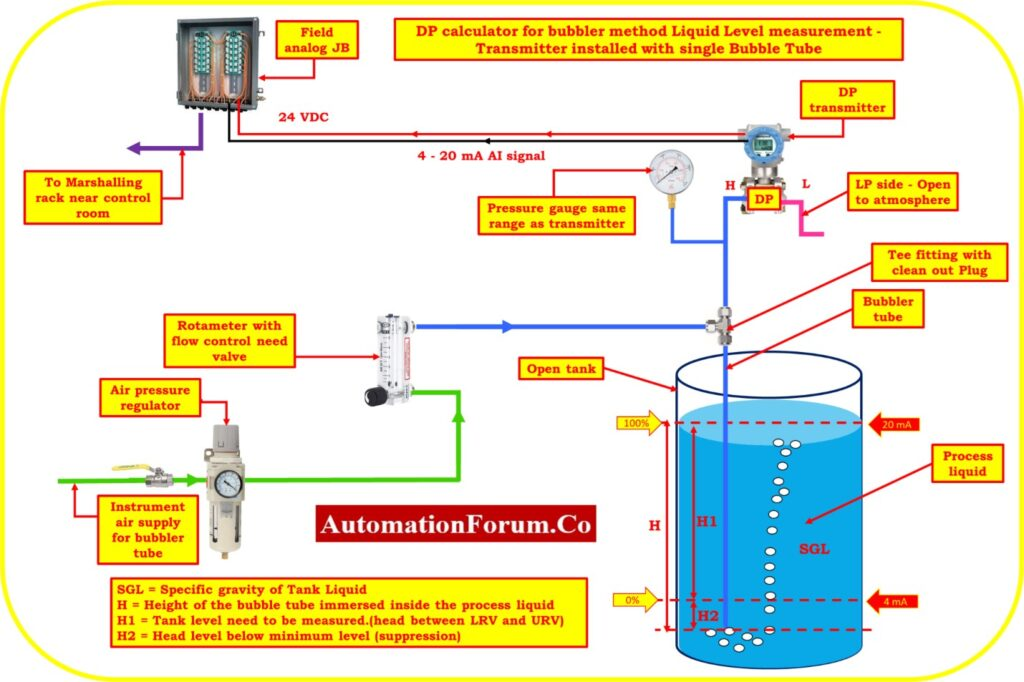# DP calculator for bubbler method Liquid Level measurement – Transmitter installed with single Bubble TubeThe level of a liquid in a tank is often measured using the bubbler method. For this technique, a bubble tube attached to a differential pressure (DP) transmitter is used. The liquid level is determined by the DP transmitter by measuring the pressure differential between the gas and liquid phases in the bubble tube. You must take the liquid density, gravity’s acceleration, and the liquid height into account when calculating the differential pressure using this approach. You may find the differential pressure value by entering these numbers into the formula.

In a variety of industrial applications, the DP calculator for the bubbler technique is a helpful instrument for precise liquid level measurement and management.

LRV = Lower-range value (a measurement that’s output is 4 mA).

URV = Upper-range value (a measurement with a 20 mA output).

SG = A liquid’s specific gravity (relative density). Water has a specific gravity of 1.00 in both traditional and SI systems.

HW= Equivalent Head level pressure of Water

SGL = Specific gravity of Tank Liquid

H = Height of the bubble tube immersed inside the process liquid

H1 = Tank level need to be measured.(head between LRV and URV)

H2 = Head level below minimum level (suppression)

Calibrated Range of the transmitter = LRV to URV

## Formula for Span value calculation

Span value of the transmitter = H1*SGL

## Formula for LRV estimation

Equivalent Head level pressure of Water (HW) at Lower range value

HW at LRV = H1*SGL = Suppression

## Formula for LRV estimation

Equivalent Head level pressure of Water (HW) at Higher range value

HW at URV = (H1 + H2)*SGL

## DP calculator

This below calculator used to calculate the Span value, LRV and URV of the transmitter for calibration in liquid level measurement